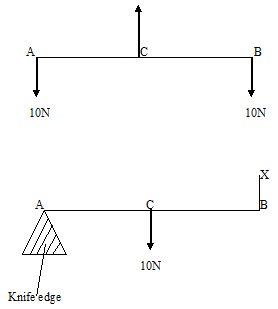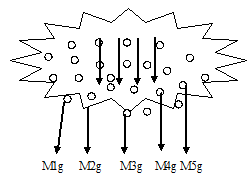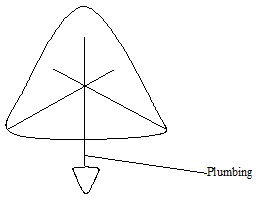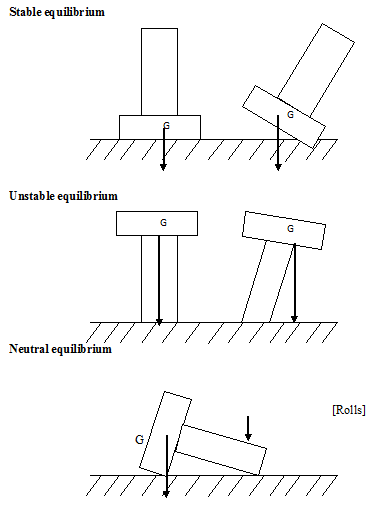# PHY3: PARALLEL FORCES

##### This unit is about Parallel forces and the center of Gravity.

PARALLEL FORCES

These are forces that act in either the same direction of opposite direction. Their resultant can be force by algebraically adding them or subtracting one from the other. However, if a body is in equilibrium, the forces acting in one direction should be equal to the forces acting in opposite direction i.e. the upward forces on the body must be equal to the downward forces.

Also the sum of clock wise moments must be equal to the sum of the anti clockwise moments. This implies that the resultant moment on the body is zero.

THIS VIDEO IS ABOUT PARALLEL FORCES

Examples

A rod of mass 0.2kg and length 2m is supported by spraining. The 3 forces are hung on the point of the rod shown below.

Find the forces Ra and RbW = mg

= 0.2 x 10 x 10

= 2ON

Solution

Sum of upward forces = sum of downward forces

RA + RB =          2 + 5 + 2 +10

RA + RB =          2 + 5 + 2 +10

RA + RB =          19 – [i]

Take moments about A, such that RA is temporarily ignored

Clockwise

[2 x 10] + 5 x [30 + 10] + [2 x 100] + [10 x 120]

20 + 200 +200 + 1200

1620NcmIf RB = 9N, then RA is 10N [using equation [i]]

In the figures below, distance AC = CB, calculate in each case the force X with which the system will keep stationary.Upward force  =         downward force

X = 10N + 10 N

X = 20N

Let distance be 2

Clockwise

10 x 2

= 20N

Anticlockwise

4 x X

= 4X

4X = 20

4x/4    =  20/4

X = 5N

COUPLE AND TOPQUE

A pair of parallel forces that are equal but opposite and acting on either sides of a pivot is called a couple e.g. turning a top or a steering wheel of a vehicle. The turning effect produced by a couple is called the torques.

The torque is often called the moment or the couple and is noted by this letter J [tau]. The tongue [tau] is the product of one of the couple and the perpendicular distance between them.F x + y = d

T = fd

T = f x [X + Y]

T = F[X + Y]

Find the tongue on a steering wheel if a couple of 100N acts on it and the radius of the wheel is 10cm

R = 10

D = 2 x 10

= 20Nm

T = fd

= 100 x 0.2

= 20Nm

To m

20cm = 0.2m

CENTRE OF GRAVITY

The centre of gravity of a body is defined as the point of application of resultant force on the body, due to the earth’s attraction on it.

The centre of gravity is therefore a point on a body where the body’s resultant weight acts.M = M1 + M2g + M3g ………..

Or

M =  mi

I = 1

A hole of a complete object is made up of a large number of tiny particles each of   tiny particles each of mass mi [ t = 1,2 3,…. N]. Each of these particles has a weight [mig]. The sum of these weights gives the total weight of each body and acts through a point inside or outside the body called its centre of gravity.

The centre of gravity of a uniform meter rule is at the 50cm mark. This can be shown by balancing a meter rule over a knife edge.

For a rectangular or square shaped uniform body the centre of gravity is at point were the diagonals meet.

For a uniform circular object, the centre of gravity is at the intersection of the line of symmetry.

A centre of gravity of a uniform triangular lamina.The following procedure is followed;-

At the 3 hole should be made at the edges of a triangular body and the body should be suspended from one of the holes on2 a clump.

From this same suspension point a plumb line should be hanging and its vertical position along the body is suspended from the other remaining holes. The point of intersection of the lines that trace the position of the plumb line is the centre of gravity of this body.

Note

Bodies which have regular shapes and uniform thickness their centers of gravity at the centre point

For example a uniform disk

Equilibrium and stability

The following conditions should be satisfied by a body in equilibrium;-

1. The resultant force acting on such a body must be zero [the total upward force is equal to the total downward force].
1. The sum of the sum of the anticlockwise moments taken about the same point
1. Objects at equilibrium will stay in one position when not disturbed by external force.

Types of stability

The centre of gravity of a body and the moment of its weight determine its equilibrium.

State equilibrium

An object which returns to its rest position after being slightly displaced is said to be in a stable equilibrium. Such a body has a broad base hence a low centre of gravity. Its centre of gravity is raised during tilting but the result tent moment brings it back to the rest position.

Unstable equilibrium

An object that falls off when slightly displaced is said to have an unstable equilibrium. Such a body has a high centre of till cause it to fall because this lowers its centre of gravity moves the centre of gravity outside the centre of the body.

Neutral equilibrium

If a body is slightly displaced, the body does not fall but rolls. The centre of gravity does not change during displacement and hence no development of resultant movement and the body is always in equilibrium.THIS VIDEO IS ABOUT THE CENTRE OF GRAVITY

TOPPLING.

The position of the centre of gravity of a body determined where she or not it topples over easily. A body topples over when the vertical line [its weight] through its centre of gravity falls outside its base.

Otherwise the body will remain stable when displaced.

Hence the stability of a body is increased by;-

1. Lowering the centre of the gravity
2. Increasing the base area [ a broadness of the base]

Applications of stability and equilibrium

1. Manufacture of racing cars
2. Manufacture of passenger vehicles likes buses where large is packed below the bus than on top of the bus.
• Construction of tall building to safe guards against earth quake
1. Large help to tractors, heavy duty tractors/ trucks and construction plants help to lower the centre of gravity of such vehicles making them more stable.

### ASSIGNMENT : PARALLEL FORCES assignmentMARKS : 10  DURATION : 1 week, 3 days

SEE ALL
•YOU